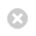Close

Mathematics

Home# Research

Research in mathematics at UCL covers many areas of modern pure and applied mathematics, including topics such as pure and applied analysis, geometry and topology, number theory, inverse problems, fluid dynamics, mathematical modelling, numerical analysis, and financial mathematics. Follow the links below to learn more about our research.# Analysis

Research areas include: spectral geometry, periodic and almost-periodic spectral problems, Toeplitz operators, spectral theory of automorphic forms, geometric scattering theory, random operators, matrix-valued operators, integrable systems, Nevanlinna theory and statistical mechanics.# Applied Analysis

The Applied Analysis group at UCL Mathematics has a broad range of research interests in asymptotic-, functional-, complex- and stochastic analysis.# Clinical Operational Research Unit

Our researchers dedicated to applying operational research, data analysis and mathematical modelling to problems in health care.# Combinatorics

Combinatorics is the branch of mathematics concerned with counting discrete structures of various types (permutations, graphs, lattice points, etc.).# Financial Mathematics

Research areas range from stochastic analysis and the theory of stochastic processes to more applied topics including the analysis and modelling of data sets.# Fluid Mechanics

The current fluid mechanics research group develops analytical and computational tools to study and the behaviour of fluids across a wide range of length scales and applications.# Geometry and Topology

The research interests of the geometry and topology group range from low-dimensional topology and geometric group theory, through algebraic geometry to symplectic geometry (and topology), gauge theory, differential geometry and geometric analysis.# Mathematical Biology

We cover a wide range of topics including analysis of the global properties of dynamical systems models in ecology, the  study of evolutionary games and evolutionary dynamics modelling in biomedicine, embryonic development systems, the biology of cancer.# Mathematical Modelling in Industry and Society

Mathematical Modelling is a key contributor in many, perhaps almost every, branch of science, engineering, technology, medicine as well as architecture, planning, commerce, arts, humanities and much more.# Mathematical Physics

Areas of research include continuum mechanics, integrable systems, general relativity and cosmology.# Number Theory

There are three main groups working on the cohomology of arithmetic groups, the properties of Shimura varieties and the arithmetic of modular and automorphic forms.# Numerical Analysis and Scientific Computing

The main focus is on the design and implementation of efficient and accurate computational methods for the approximation of solutions to partial differential equations.